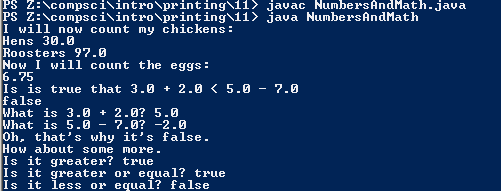# Assignment #11 and NumbersAndMath

## Code

```      /// Name: Jason Kim
/// Period: 7
/// Program Name: NumbersAndMath
/// File Name: NumbersAndMath.java
/// Date Finished:9/10/15

public class NumbersAndMath
{
public static void main (String[] args)
{
//prints "I will now count my chickens:"
System.out.println("I will now count my chickens:");

//prints the string and the calculated number
System.out.println("Hens " + (25.0+30.0/6.0));
//prints the string and the calculated number
System.out.println("Roosters " + (100.0-25.0*3.0%4.0));
//prints statement
System.out.println( "Now I will count the eggs:");
//prints the calculated number
System.out.println(3.0+2.0+1.0-5.0+4.0%2.0-1.0/4.0+6.0);
//prints the string
System.out.println( "Is is true that 3.0 + 2.0 < 5.0 - 7.0");
//false statement which prints out false
System.out.println( 3.0 + 2.0 < 5.0-7.0);
//prints string and the calculated number
System.out.println( "What is 3.0 + 2.0? " + ( 3.0 + 2.0 ));
//prints string and the calculated number
System.out.println( "What is 5.0 - 7.0? " + ( 5.0 - 7.0 ));
//prints the string
System.out.println( "Oh, that's whiy it's false." );
//prints the string
System.out.println( "How about some more." );
//prints the string and true because the statement is true
System.out.println( "Is it greater? " + ( 5.0> -2.0 ) );
//prints the string and true because the statement is true
System.out.println( "Is it greater or equal? " + ( 5.0>= -2.0) );
//prints the string and false because the statement is false
System.out.println( "Is it less or equal? " + ( 5.0<= -2.0 ) );
}
}
```

### Picture of the output